# The network effect: understanding Uber by studying meerkats

Ecology (and systems of differential equations) can tell us a lot about the network effect behind apps like Uber and Facebook.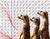by Mojan Benham

A year ago

## Two-line summary

In Andrew Chen's "The Cold Start Problem", he draws an analogy between network-based apps like Uber and the ecology of meerkats as discussed in his uWashington classes. Having taken this course at UW myself, here I revisit the curriculum to demonstrate how solving a system of differential equations is a familiar way to understand the network effect.

Basic understanding of differential equations and eigenvalues is required.

## A brief definition of the network effect

The network effect describes how the value of a system changes as a function of the size of its user base. The success of companies like Facebook and Tinder relies on fostering the health of these networks as the products grows. This may consist of sets of interconnected users or a multi-sided marketplace, with Uber having drivers and passengers or Airbnb connecting hosts to guests.

In "The Cold Start Problem" (which I highly recommend), author Andrew Chen recalls the challenges of maintaining this balance at Uber: too few drivers would leave passengers frustrated with wait times, while saturating drivers would result in too much competition. He defines the theory of network effects as follows: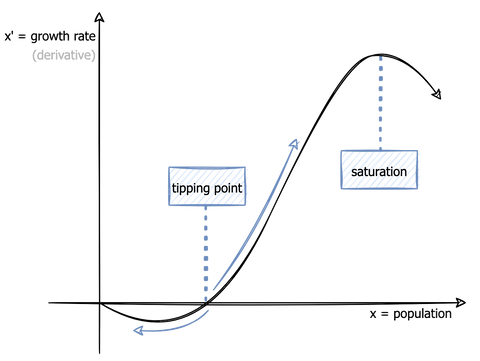The x-axis describes the size of the population x, while the y-axis describes the growth rate of the population, otherwise known as the rate of change or derivative x'. The population has a tipping point below which the network collapses and above which the network may grow to its carrying capacity (saturation).

## Meerkats and Uber: what do they have in common?

To further explain this theory, Chen introduces an analogy on the social tactics of meerkats, who gather in gangs to warn each other about potential threats. A meerkat gang smaller than the tipping point is too few to protect itself from its natural predator, hawks. This leaves them vulnerable to be preyed on, thus decreasing the size of the population only to leave it even more vulnerable, and the cycle continues until extinction. However, above the tipping point, the size of the gang is strong enough to fend off predators and grow up to the carrying capacity, which is a population cap imposed by limited food resources.

During my graduate work at our shared alma mater, I took a course on differential equations where many of the applications were related to said predator-prey models. If x is the meerkat population, then x' represents the rate at which the population size changes for this species. We may also define hawks as y and y' respectively. These derivatives give us a system of differential equations that may be solved for thresholds like the tipping point and therein allow us to understand the network conditions under which both species could coexist. But what does this have to do with Uber?

Well, you can let x and y be any interdependent set of variables like drivers and passengers (Uber), hosts and guests (Airbnb), content creators and viewers (YouTube), supplier and consumers (Wayfair), etc. The math we've come to understand about predator and prey can be applied to the networks we see prevalent in the tech world.

Let's now shift our focus to solving and interpreting systems of differential equations in order to demonstrate how the conditions for a successful network are determined.

## Introduction to phase planes

The network effect graph above is an example of a one-dimensional phase diagram: a visualization of the characteristics of one variable, x, and its rate of change x'. There are essentially two components in this diagram:

1. The points at which the growth rate, x', changes signs (aka x' = 0) called equilibrium points.
2. The arrows that indicate the sign changes of x'

In the case above, the equilibrium points include the point at ﻿x = 0﻿ and the tipping point.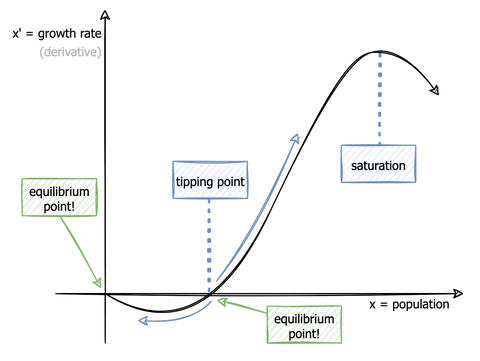With this updated diagram, we can introduce the concept of stability. The stability of an equilibrium point describes whether values of x go towards it (stable) or away from it (unstable).
The tipping point is unstable because a population size below it has a negative x' and the population collapses to zero, while a population above this threshold has x' > 0 and the population grows toward the carrying capacity. The point at x = 0 is stable because any initial condition between it and its adjacent equilibrium point (the tipping point in this case) will ultimately lead to the population moving toward zero.
This example had only one variable, but what if the system has two variables like hawks and meerkats, or drivers and passengers? In the two-dimensional case we move from a phase diagram to a phase plane.
Let's use a simple example of a system of equations where x is Uber drivers and y is Uber passengers (in units of millions). The following model is purely hypothetical and is chosen because it serves as a simple example:
$x'=3x-2xy-x^2$$y'=2y-xy-y^2$
These are a bit trickier to sketch by hand, so you can use any online phase plane plotter to produce the graph shown below. I use this one. Note that since populations can only exist on a range of positive numbers, only the first quadrant is considered valid.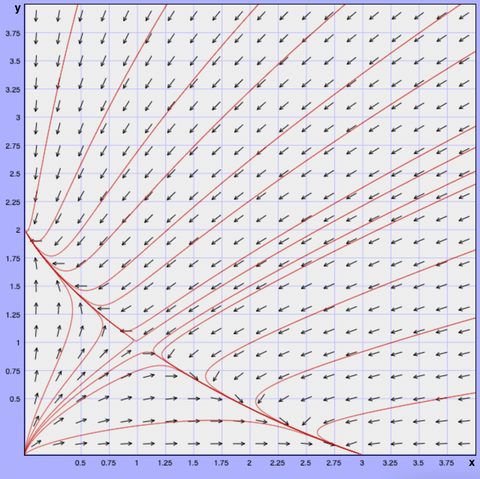The x and y axes are the respective driver and passenger populations, and the arrows show how these populations change (x' and y') just as in our 1d example. Don't worry about understanding this graph just yet, but note that it's similar practice to how we interpreted the one-dimensional case above. The origin, for example, appears to be unstable because all arrows lead away from it.
What ﻿is important is that with these concepts, we can lay out the skeleton for understanding the network effect:
1. Define the population variable(s), x (and y if it's a multi-sided network)
2. Derive a model of the population variables as a system of differential equations
3. Find the equilibrium points of the system
4. Determine the stability of the equilibrium points
5. Draw the phase diagram
6. Interpret the phase diagram to determine the conditions under which the network can succeed

Note that in practice, 2. is not straightforward. Deriving a model for the complexities of a network requires a great deal of research and the work of many skilled data scientists and even then may not be possible, so I recognize the theoretical scope of this post.

## Why do networks collapse below the tipping point?

In the meerkat example, populations under the tipping point are too small to defend themselves, so unless they grow beyond that point they will eventually be hunted until extinction. Similarly, consider using a messaging app like WhatsApp while most of your friends are on Facebook Messenger. Eventually some of your WhatsApp friends will leave for Messenger and you will have no one to talk to, so you also make the switch. This leaves any remaining WhatsApp users in your network to choose between deleting the app or following suit and the WhatsApp network collapses.

Let's now describe "collapse" in mathematical terms: two adjacent equilibrium points in a one-dimensional phase diagram cannot have the same stability (i.e. if x is moving toward one equilibrium point, it's implied that it must be moving away from the other). In our very first example, this means that any initial population between ﻿x = 0 and its adjacent equilibrium point (the tipping point in our case) will attract the population size toward zero. For this reason, the network is at risk of collapsing at any point below the tipping point, unless it can grow past it to reach x' > 0.

Note that although in this case there is only one, networks can have many tipping points depending on the complexity of the model.

## Solving for equilibrium points in a system of differential equations

Let's now return to our two-dimension example and solve for the equilibrium points. Recall that x is the number of Uber drivers and y is the number of Uber passengers (in millions) and that the system is defined as follows:

$x'=3x-2xy-x^2$$y'=2y-xy-y^2$

To solve for the equilibrium points, we essentially want to find the points where the derivatives change signs, which occurs at x' = 0 and y' = 0. For x' = 0:

$x'=3x-2xy-x^2$$0=3x-2xy-x^2$$0=x(3-2y-x)$

Thus x = 0 and x = 3 - 2y. For y' = 0:

$y'=2y-xy-y^2$$0=2y-xy-y^2$$0=y(2-x-y)$

This gives us y = 0 and y = 2 - x. The result is four equilibrium points:

1. x = 0, y = 0
2. x = 0, y = 2   since y = 2 - x
3. x = 3, y = 0   since x = 3 - 2y
4. x = 1, y = 1   since y = 2 - x = 2 - (3 - 2y)

Now that we're aware of four equilibrium points (circled in black), let's reinspect the phase plane.Notice that the arrows lead toward (0,2) & (3,0) and away from (0,0) & (1,1) and while it may be temping to make conclusions about stability based on visual inspection alone, there is a mathematical way to conduct this analysis.

## Conducting stability analysis

In order to determine the stability of the equilibrium points, we must find the eigenvalues of the system. If either eigenvalue in an eigenvalue pair is positive and real, the point is unstable. However if both eigenvalues are negative and real, then the point is stable.

Recall from linear algebra that to find the eigenvalues, we must first construct the Jacobian. Let x' = u and y' = v. Then the Jacobian is defined as such:

$J=\begin{pmatrix}\frac{du}{dx}&\frac{du}{dy}\\\frac{dv}{dx}&\frac{dv}{dy}\end{pmatrix}$$J=\begin{pmatrix}3-2x-2y & -2x\\-y&2-2y-x\end{pmatrix}$

Now we evaluate the Jacobian matrix at each of the equilibrium points. Note: a useful property is that when the matrix is upper triangular, lower triangular or diagonal, the eigenvalues can simply be read off of the diagonal. Starting with the origin:

$J=\begin{pmatrix}3-2x-2y&-2x\\-y&2-2y-x\end{pmatrix}$$J(0,0)=\begin{pmatrix}3&0\\0&2\end{pmatrix}$

This is diagonal matrix, so we read off eigenvalues 3 & 2. Since both are positive and real, the origin is unstable. Moving on to (0,2):

$J=\begin{pmatrix}3-2x-2y&-2x\\-y&2-2y-x\end{pmatrix}$$J(0,2)=\begin{pmatrix}-1&0\\-2&-2\end{pmatrix}$

This is a lower triangular matrix so we read the eigenvalues off of the diagonal as -1 and -2. Both eigenvalues are negative and real, therefore (0,2) is stable. Now for (3,0):

$J=\begin{pmatrix}3-2x-2y&-2x\\-y&2-2y-x\end{pmatrix}$$J(3,0)=\begin{pmatrix}-3&-6\\0&-1\end{pmatrix}$

This is an upper triangular matrix so we read the eigenvalues off of the diagonal as -3 and -1. Again, they are both negative and real so ﻿(3,0) is stable﻿. And finally, for (1,1):

$J=\begin{pmatrix}3-2x-2y&-2x\\-y&2-2y-x\end{pmatrix}$$J(1,1)=\begin{pmatrix}-1&-2\\-1&-1\end{pmatrix}$

In this case we cannot read off the diagonal, so we'll compute the determinant and solve:

$\begin{vmatrix}-1-\lambda&-2\\-1&-1-\lambda\end{vmatrix}\rightarrow(-1-\lambda)^2-2=0\rightarrow\lambda=-1\pm\sqrt{2}$

There is one positive eigenvalue and one negative eigenvalue, so this equilibrium point (1,1) is unstable. This confirms what we observed in the phase plane; the stable points are the ones where arrows flow toward them and the unstable nodes have arrows moving away.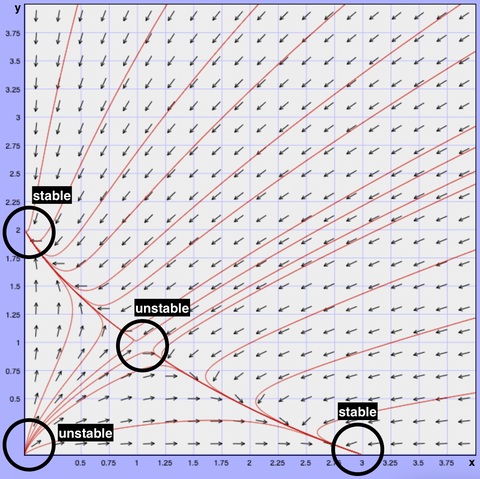## Interpreting the math

This process is useless without interpretation, so let's now discuss what these results mean in terms of the network effect. In this particular model, the only stable points exist where there are no drivers (x = 0) or no passengers (y = 0) thus there are no conditions under which drivers and passengers can exist harmoniously.

I've drawn a green line on the phase plane below to divide the graph into two sections. For all values of x and y to the left of the green line, all arrows point to drivers completely leaving the platform due to the attraction to stable point at (0,2). To the right of the line, all arrows move toward collapsing to the point of no passengers at the stable point at (3,0).

In mathematical terms, the set of initial conditions that all move toward a particular equilibrium point is called a basin of attraction. As in, all points to the right of the green line are part of one basin of attraction. The line itself is called a separatrix.If this model were the reality of our driver/passenger scenario, the result is quite dire because there is no "tipping point" at which the a healthy network can be achieved. Obviously this is not the case for businesses like Uber and Lyft, where a stable point would have to exist among positive values in the quadrant. For example, if our model were such that (1,1) were actually stable instead of unstable, then at that population size, ﻿x﻿ and ﻿y ﻿could exist without collapsing.

## Closing thoughts

Like many students at some point, I found myself in a lecture hall questioning why I was paying someone to teach me about meerkats. It's fascinating to reflect on how intuitively the dynamics of ecology explain the network phenomena I've experienced both as a user and builder of networks. Evaluating systems of differential equations removes the mystery of why some apps seem to lose momentum and collapse over time.

To learn more, Andrew Chen's book reads like a novel and provides diverse case studies that dive deep on the topic of network effects. For a mathematical understanding, try an introductory course to dynamical systems - I took AMATH 502 in my time at uWashington if you're interested.

As always, would love to hear your thoughts and feedback in the comments below.

## 1 comment

• This was such a fascinating read. Very nicely written and explained

Niki on